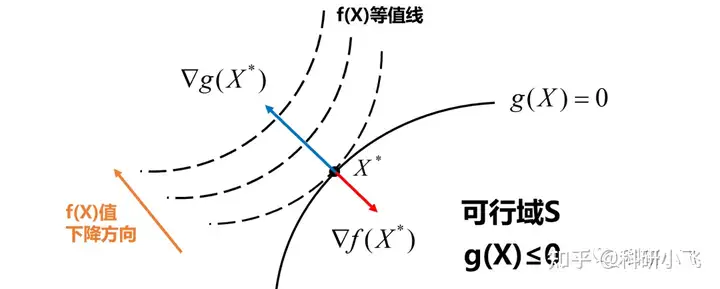1. 构造拉格朗日函数 $L(x,y,\dots,\lambda) = f(x,y,\dots) + \lambda g(x,y, \dots)$，其中 $f(x,y,\dots)$ 是原问题目标函数，$g(x,y, \dots)$ 是约束条件。
2. 求解方程组 $\frac{ \partial L }{ \partial x }=0,\frac{ \partial L }{ \partial y }=0,\dots,\frac{ \partial L }{ \partial \lambda }=0$，得到所有可能的极值点 $(x,y,…,λ)$
3. 将极值点代入目标函数 $f(x,y,\dots)$，比较大小，得到最大值和最小值。

1. 构造拉格朗日函数$L(x,y,\lambda) = f(x,y) + \lambda g(x,y)$，其中 $g(x,y) = x + y - 1$ 是约束条件转换而来。
2. 将偏导置零得到方程组 $\partial L/\partial x = 2x + \lambda = 0,\partial L/\partial y = 2y + \partial = 0,\partial/\partial \lambda L = x + y - 1 = 0$
3. 解得  $\lambda = -2,x = y = 1/2$，由于只有一组解，所以即为全局最优解。

\begin{aligned} \min \quad &f(x)\\ s.t. \quad &g_{i}(x)\leq 0,i=1,\dots,m\\ &h_{j}(x)=0,j=1,\dots,n \end{aligned}

$L(x,\lambda,\mu)=f(x)+\sum_{i=1}^n\lambda_{i}g_{i}(x)+\sum_{j=1}^n\mu_{j}h_{j}(x)$

• 稳定性条件 ：$\nabla L(x^\star,\lambda^\star,\mu^\star)=0$
• 原始可行性：$g_{i}(x^\star)\leq 0;h_{j}(x^\star)= 0$
• 对偶可行性：$\lambda^\star_{i}\geq 0$
• 互补松弛可行性：$\lambda_{i}^\star g_{i}(x^\star)=0$

## 对偶可行性$x^\star$ 若是一个最优解，在 $x^\star$ 处，$g(x)$$f(x)$ 的梯度方向应该共线且相反，由稳定性条件可得
$\nabla L(x^\star,\lambda^\star,\mu^\star)=\nabla f(x^\star)+\sum_{i}\lambda_{i}\nabla g_{i}(x^{\star})=0\implies \lambda\nabla g(x)=-\nabla f(x)$$\lambda g(x)$$f(x)$梯度方向也相反，所以这里$\lambda\geq 0$否则不满足$g(x)$$f(x)$

$f(x^\star)= g(\lambda^\star,\mu^\star)\leq f(x)+\sum_{i=1}^n\lambda_{i}^\star g_{i}(x^\star)+0$

## 松弛互补可行性

$x^\star$$g_{i}(x^\star)< 0$时，即正好满足约束，此时约束并不起作用，所以$\lambda_{i}^\star=0$
$x^*$$g_{i}(x^\star)=0$ 时，即可以转换为等号约束条件。

# 拉格朗日对偶问题

$L(x,\lambda,\mu)$ 看作是关于 $\lambda,\mu$ 的函数，求其最小值为拉格朗日对偶函数：

$\theta(x)=\min_{x} L(x,\lambda,\mu)$

\begin{aligned} \max_{\lambda,\mu;\lambda\geq 0} \quad &\theta(\lambda,\mu)\\ s.t. \quad &\lambda\geq 0 \end{aligned}

$\max_{\lambda,\mu;\lambda\geq 0}\theta(\lambda,\mu)\leq \min_{x} \max_{\lambda,\mu;\lambda\geq 0}L(x,\lambda,\mu)$

$\max_{\lambda,\mu;\lambda\geq 0}\theta(\lambda,\mu)= \min_{x} \max_{\lambda,\mu;\lambda\geq 0}L(x,\lambda,\mu)$